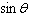Name:    Chapter 4 Review

True/False
Indicate whether the statement is true or false.

1.

An angle in standard position has an initial arm located on the x-axis.

2.

Exactly three angles between 0° and 360° have the same value for the sine ratio.

3.

The CAST rule identifies positive primary trigonometric ratios for angles.

4.

The reciprocal trigonometric ratios are cosecant, secant, and cotangent.

5.

When two solutions exist for given information in a trigonometry problem, it is referred to as the arbitrary case.

6.

Solving a problem in three dimensions usually involves solving two problems that are each two-dimensional problems.

7.

The expression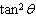can also be written as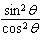.

Multiple Choice
Identify the choice that best completes the statement or answers the question.

8.

For the given triangle, tan A is equal to which expression?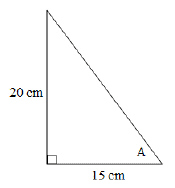a.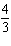c.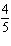b.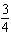d.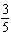9.

Which statement regarding sin 30° is true?
 a. sin 30° = cos 60° c. sin 30° = sin 150° b. sin 30° =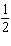d. all of the above

10.

What is the measure of ÐB in the triangle shown?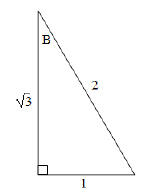a. 30° c. 60° b. 45° d. 90°

11.

What reference angle should be used for an angle of 300° in standard position?
 a. 30° c. 60° b. 45° d. 300°

12.

In which quadrants are the tangent ratios negative values?
 a. first and second c. third and fourth b. second and third d. second and fourth

13.

Which set of angles has the same terminal arm as 40°?
 a. 400°, 760°, 1120° c. 130°, 220°, 310° b. 220°, 400°, 580° d. 80°, 120°, 200°

14.

The point (3, 4) is on the terminal arm of ÐA.Which is the set of exact primary trigonometric ratios for the angle?
 a.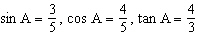c.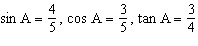b.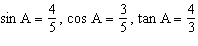d.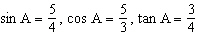15.

The point (3, –5) is on the terminal arm of ÐD.Which is the set of exact primary trigonometric ratios for the angle?
 a.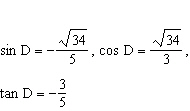c.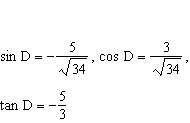b.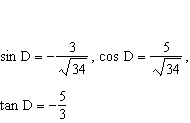d.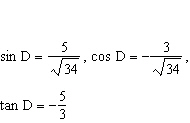16.

What is the exact value for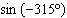?
 a.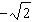c.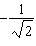b.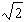d.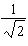17.

Which pair of angles between 0° and 360° satisfies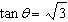?
 a. –60° and –240° c. 120° and 240° b. 60° and 240° d. 300° and 240°

18.

What is the measure of ÐA, if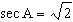and ÐA is located in the first quadrant?
 a. 70.5° c. 22.5° b. 45° d. 135°

19.

Which of the following is the correct expression for csc A?
 a.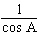c.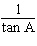b.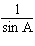d.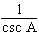20.

Determine two angles between 0° and 360° that have a cosecant of –2.
 a. 30° and 150° c. 330° and 210° b. –30° and –150° d. 240° and 300°

21.

In solving a triangle, which of the following situations requires the ambiguous case to be considered?
 a. two sides and one opposite angle c. three sides b. two sides and the contained angle d. one side and two angles

22.

Which strategy would be best to solve for x in the triangle shown?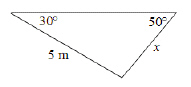a. sine law c. primary trigonometric ratio b. cosine law d. none of the above

23.

Determine the length of x, to the nearest tenth of a metre.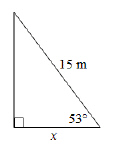a. 24.9 m c. 12.0 m b. 9.0 m d. 18.8 m

24.

Determine the length of x, to the nearest tenth of a centimetre.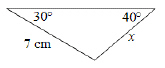a. 5.4 cm c. 9.0 cm b. 7.9 cm d. 6.2 cm

25.

Determine the length of x, to the nearest tenth of a metre.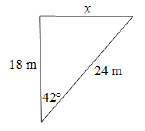a. 257.9 m c. 15.9 m b. 39.3 m d. 16.1 m

26.

Determine the measure of x, to the nearest tenth of a degree.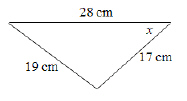a. 48.4° c. 20.8° b. 41.6° d. 35.5°

27.

Determine the length of x, to the nearest tenth of a kilometre.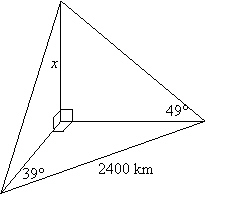a. 2100.0 km c. 2400.0 km b. 1737.5 km d. 990.9 km

28.

What does the expression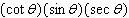simplify to?
 a.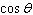c. 1 b.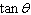d.